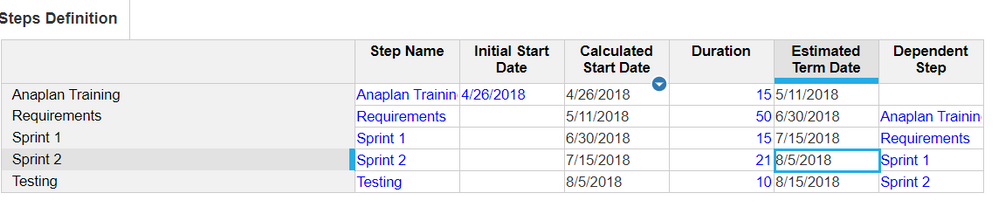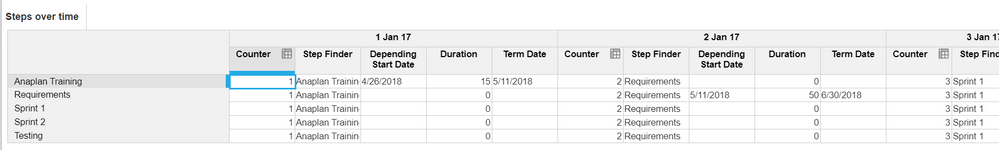# {Trick] Previous on regular list / sequential calcs using time as daysHi everyone,

I've been talking about this in the forums a few times and recently @gaurav_sharma-bng  told me he couldn't get it to work so here's a quick topic on how to do this.

Used for:

Doing sequential calculations in Anaplan or doing previous on a regular list

Disclaimer:

as we're using time as days, it's quite a heavy solution. So use it with great caution.

Example:

I came accross this when I started Anaplan 5 years ago (work anniversary this week) and it was one of the first complex technical tricks I solved. I wanted to do some kind of Gantt logic where the date of step is dependent on the date of the previous step. Years later, I mostly use this to do SEQUENTIAL CALCULATIONS.

You can see here, on the Dependent Step line item, I'm linking the steps together. We often do that when doing custom timescale. You'll be able to lookup on this line item.I'll explain the formulas once you get the calculation module, as below:You can see this module has the "Steps" list + the time, in daily(use a one year only time range).

The whole thing here is to be able to identify every step with a day: you can see training = 1 jan, Requirements = 2 Jan etc

For this, the counter is used, it is a simple formula. Note the subsidiary view, as thie line item does't need the steps dimension. This method is usually heavy so you will want to save space.

`1+PREVIOUS(COUNTER)`

Now in Step Finder, you can use FINDITEM formula to identify the step that has this code:

`FINDITEM(Project Steps, TEXT(Counter))`

In my case, I went for extremely simple codes for the Steps list : 1,2,3,4,5,6. You can use more complex codes, as long as there is an increment that you can match as the day increases.

ps: we shouldn't nest formulas within (especially) FINDITEM, avoid it if you have the space

From there, it is actually done. Depending Start Date formula is:

```IF Step Finder = ITEM(Project Steps) THEN
IF ISBLANK(Steps Definition.Dependent Step) THEN
Steps Definition.Initial Start Date
ELSE
PREVIOUS(Term Date[LOOKUP: Steps Definition.Dependent Step])
ELSE BLANK```

The first line is used to identify if the Step item of the list is the same as the Step found with the FINDTEM. Otherwise, we don't want to do anything. Then I'm simply checking if the step is the first, in which case I'll take the initial start date, ELSE I take the previous term date. This is where the PREVIOUS on a normal list if doable. as it is mixed with time.

Duration formula :

`IF Step Finder = ITEM(Project Steps) THEN Steps Definition.Duration ELSE 0`

Term Date formula:

`IF Step Finder = ITEM(Project Steps) THEN Depending Start Date + Duration ELSE BLANK`

Now you can simply bring back the correct dates to the Steps definition module by SELECTing the Time.All Periods

`Steps over time.Depending Start Date[SELECT: TIME.All Periods]`

I hope this is clear

Conclusion:

This is a complex trick that is heavy on the space and performance: avoid it if you can. However sometimes you just can't and it is very useful. I've used it in several projects to do sequential calculations such as supplier allocations, allocation of a value to tiers (for commission), dependent dates etc.

Beware that it is not unlimited: as you are using days, you only have 365 sequences in your calc.

Tagged: